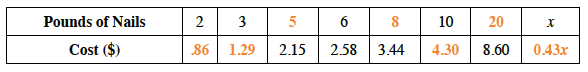Home > ACC6 > Chapter cc24 > Lesson cc24.2.3 > Problem4-50

4-50.

Complete the proportion table below and graph the results. 4-50 HW eTool (Desmos). Homework Help ✎

 Pounds of Nails $2$ $3$ $6$ $10$ $x$ Cost (\$) $2.15$ $2.58$ $3.44$ $8.60$

If $6$ pounds of nails costs $\2.58$, how much does $3$ pounds of nails cost?

$\frac{6}{2.58}\left(\frac{?}{?}\right)=\frac{3}{\textit{x}}$

Solve for $x$.

$x=\1.29$
Now set up proportions for all other values in the table.

1. What is the unit rate (price) of the graph?

The table has been completed for you. The unit rate is the cost for one pound of nails.One way to do this is to divide the cost for two pounds of nails in half.

2. What does the unit rate (price) tell you about the cost of the nails?

The unit rate (price) tells you how much nails cost per pound.

Complete the table in the eTool below to graph the results.
Click the link at right for the full version of the eTool: CC2 4-50 HW eTool